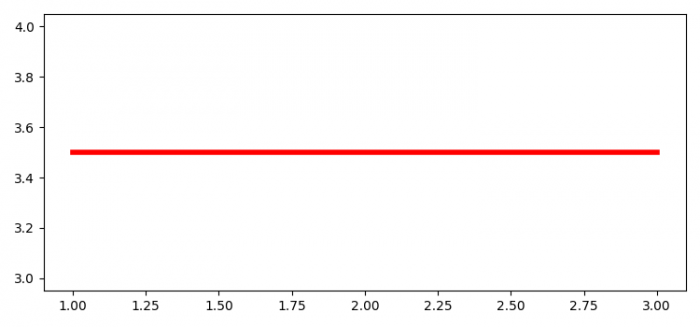# How to reuse plots in Matplotlib?

To reuse plots in Matplotlib, we can take the following steps −

• Set the figure size and adjust the padding between and around the subplots.
• Create a new figure or activate an existing figure using figure() method.
• Plot a line with some input lists.
• To reuse the plot, update y data and the linewidth of the plot
• To display the figure, use show() method.

## Example

from matplotlib import pyplot as plt

plt.rcParams["figure.figsize"] = [7.50, 3.50]
plt.rcParams["figure.autolayout"] = True

line, = plt.plot([1, 3], [3, 4], label="line plot", color='red', lw=0.5)

line.set_ydata([3.5])
line.set_linewidth(4)

plt.show()

## Output